# Angular Acceleration

## Angular acceleration

• Angular acceleration is the rate of change of angular velocity with respect to time. Thus for rigid body rotating about a fixed axis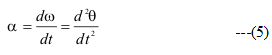• Unit of angular acceleration is radian /sec2 and it is a vector quantity like Angular velocity
• Now Let's derive it for rotation around fixed axis
• Consider a rigid body of arbitrary shape rotating about a fixed axis through point O and perpendicular to the plane of the paper as shown below in the figure-1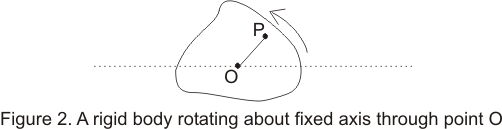• while the body is rotating each and every point in the body moves in a circle with their center lying on the axis of rotation and every point moves through the same angle during a particular interval of time
• Consider the position of a particle say ith particle at point P at a distance ri from point O and at an angle θi which OP makes with some reference line fixed in space say OX as shown below in the figure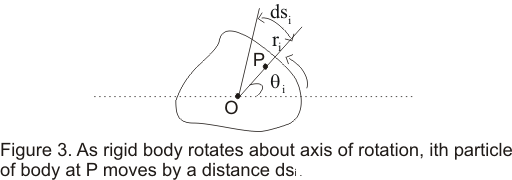• If particle moves an small distance dsi along the arc of the circle in small amount of time dt then
dsi=vidt ----(1)
where vi is the speed of the particle
• dθ is the angle subtended by an arc of length dsi on the circumference of a circle of radius ri,so dθ( in radians) would be equal to the length of the arc divided by the radius
i.e.
dθ=dsi/ri =vidt/ri ----(2)
• distance dsi would vary from particle to particle but angle dθ swept out in a given time remain same for all the particles i.e. if particle at point P moves through complete circle such that
Then all the other particles of the rigid body moves through the angular displacement dθ=2π
• Now We already know about angular velocity is given by ω=dθ/dt ----(3)
• Putting equation (3) in equation (2) we find
vi=ri(dθ/dt) =riω ---(4)
This shows that velocity of ith particle of the rigid body is related to its radius and the angular velocity of the rigid body
• Now angular acceleration can be written as
$\alpha = \frac {d \omega}{dt}$
Now from equation 3
$\alpha = \frac {d}{dt} \frac {d \theta}{dt} = \frac {d^2 \theta}{dt}$
• Angular acceleration holds not only for that rotating rigid body but also for the each and every particle of that body
• Differentiating equation (4) w.r.t to t we find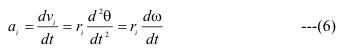where ai=ait=riα is the tangential component of linear acceleration of a point at a distance ri from the axis
• The above equation is important as it shows the relation ship the tangential components of linear acceleration of a point and angular acceleration of a point
• Each particle in the rigid body also has the radial linear acceleration component v2/r ,which can also be expressed in terms of an of angular velocity i.e.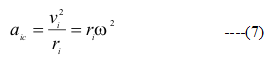and this acceleration ac pointing inwards towards the radial line is also known as centripetal acceleration
• So total acceleration of an point in the rotation can be expressed as.
$a = \sqrt {a_c^2 + a_i^2}$

Question 1. A wheel starts from rest and spins with a constant angular acceleration. As time goes on the acceleration vector for a point on the rim:

a. increases in magnitude but retains the same angle with the tangent to the rim
b.increases in magnitude and becomes more nearly radial
c. increases in magnitude and becomes more nearly tangent to the rim
d. decreases in magnitude and becomes more nearly radial
Solution
Since angular acceleration is constant,Tangential acceleration is constant

Since angular velocity increase with time,Radial acceleration increase with time

So resultant acceleration increase with time and becomes more radial as time passes

Question 2.
Two wheels are identical but wheel B is spinning with twice the angular speed of wheel A. The ratio of the magnitude of the &radical acceleration of a point on the rim of B to the magnitude of the radial acceleration of a point on the rim of A is:

a. 4
b. 2
c. 1/2
d. 1/4

Solution.
For wheel A
Radial acceleration of A =r* (ω)2
For wheel B
Radial acceleration of B=r* (2ω)2=4r* (ω)2

Question 3. For a wheel spinning with constant angular acceleration on an axis through its center, the ratio of the speed of a point on the rim to the speed of a point halfway between the center and the rim is:
a 2
b 1/2
c 4
d 1/4

Solution
At rim
$v=r \omega$
At point between the center and rim
$v=\frac {r}{2)} \omega$
Ratio =2